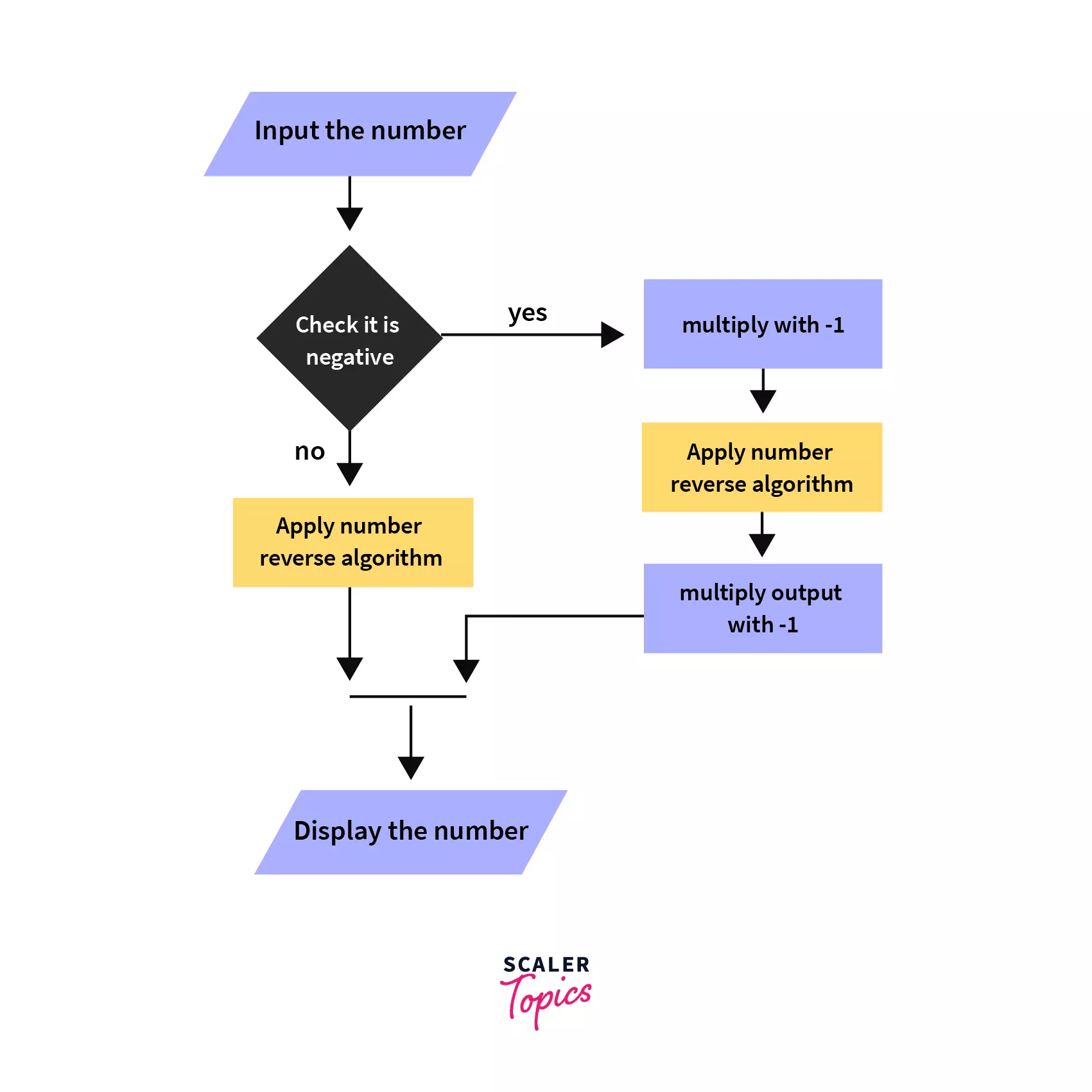C Program to Reverse a Number

Learn how to reverse a number in C with some examples

In programming, we can display the reversed number by printing all its digits from the rightmost digit to the left-most integer. But, to retain it as a number, we apply some math to store the value at each iteration.

Eg: Given Input is 2345. The required output is 5432. Let us assume our reversed number(rev_num) is 0. For every digit in 2345, we follow two simple steps.

Step 1: Multiply the rev_num with 10 and add the digit which we extracted.

Step 2: Equate rev_num with the obtained result

After 4 iterations, rev_num contains 5432 which is our required result.

Digit ExtractedMultiplication with 10
50 * 10 + 5 = 5
45 * 10 + 4 = 54
354 * 10 + 3 = 543
2543 * 10+2 = 5432

Table 1: Reverse 2345 step-by-step

Algorithm to Reverse a Number in C

1. Initialize rev_num = 0
2. For every digit in the number do step 3
3. rev_num = rev_num * 10 + digit
4. Output the rev_num

How to Implement in C Programming

We use 2 arithmetic operators namely Modulo and division operator to achieve this task

1. Modulo Operator (%) provides the remainder. Eg: 2345 % 10 = 5
2. Division Operator (/) provides the quotient. Eg: 2345 / 10 = 234

Try the above approach before snipping into the code below.

Program to Reverse a Number in C

Iterative Method of Reversing a Number Using C

#include<stdio.h>
int main(){
int n=23450;
int rev_num = 0;
while(n!=0){
rev_num = rev_num * 10 + n%10;
n = n/10;
}
printf("%d", rev_num);
}

Explanation:

1. The Terminating condition (n!=0) stops execution as it denotes that we read all digits in our number.
2. Statement rev_num = rev_num *10+ n%10 updates rev_num with partial reversed value. (Refer Table 1 for partial reversed values)

Recursive Implementation of Reversing a Number Using C

#include<stdio.h>
int reverse(int n,int k){
if(n==0)
return k;
return reverse(n/10,n%10+k*10);
}
int main(){
int n=23450;
printf("%d",reverse(23450,0));
}

Explanation:

1. In The recursive function reverse(n,k), k represents the rev_num variable in iteration code.
2. When n reaches 0, we return k since we computed our output by this time.
3. In other cases, we call reverse(n/10, n%10+k*10) which calls functions recursively with updated n, k values.
Iterationn-valuek-valuereturn value
023450reverse(2345,0)
12345reverse(234,5)
22354reverse(23,54)
32543reverse(2,543)
405432As n=0, Instead of calling reverse function we return k which is 5432

Issue with Negative Number Inputs

If the number is negative, we need to multiply it with -1 to make it positive. This positive number has to be reversed first and multiplied again with -1 to obtain its negative representation. We cannot directly apply the above algorithm directly as some compilers ignore the negative numbers and give wrong results. It is due to the change in binary representation.Analysis of Algorithm

Time Complexity

While loop / recursive function executes n times for a n-digit number. No. of digits in a number k is given by computing $log_{10}(k)$. Therefore, time complexity is $O(log_{10}(k))$.

Space Complexity

We are not using any extra space for storing the digit other than the int variable. Hence, Space complexity is O (1).

More Implementations

Representing Number as Array:

• Each digit is set to store in the array. Read the number directly into an array of characters or use a loop to store each digit from the number.
• Reverse the array using strrev() in the string.h header file.
• Inputs having trailing zeroes should be discarded as they are no longer worth.